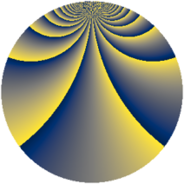# Properties

 Label 1152.3.biLevel $1152$ Weight $3$ Character orbit 1152.bi Rep. character $\chi_{1152}(113,\cdot)$ Character field $\Q(\zeta_{24})$ Dimension $752$ Sturm bound $576$

# Learn more

## Defining parameters

 Level: $$N$$ $$=$$ $$1152 = 2^{7} \cdot 3^{2}$$ Weight: $$k$$ $$=$$ $$3$$ Character orbit: $$[\chi]$$ $$=$$ 1152.bi (of order $$24$$ and degree $$8$$) Character conductor: $$\operatorname{cond}(\chi)$$ $$=$$ $$288$$ Character field: $$\Q(\zeta_{24})$$ Sturm bound: $$576$$

## Dimensions

The following table gives the dimensions of various subspaces of $$M_{3}(1152, [\chi])$$.

Total New Old
Modular forms 3136 784 2352
Cusp forms 3008 752 2256
Eisenstein series 128 32 96

## Trace form

 $$752q + 8q^{3} - 12q^{5} + 4q^{7} - 8q^{9} + O(q^{10})$$ $$752q + 8q^{3} - 12q^{5} + 4q^{7} - 8q^{9} + 12q^{11} - 4q^{13} + 16q^{19} - 8q^{21} + 12q^{23} - 4q^{25} + 104q^{27} - 12q^{29} + 8q^{31} - 16q^{33} - 16q^{37} - 184q^{39} - 12q^{41} + 4q^{43} - 8q^{45} + 80q^{51} + 16q^{55} - 8q^{57} + 12q^{59} - 4q^{61} + 16q^{63} - 24q^{65} + 4q^{67} - 8q^{69} - 16q^{73} - 92q^{75} - 12q^{77} - 1428q^{83} - 104q^{85} - 440q^{87} + 16q^{91} + 28q^{93} + 24q^{95} - 8q^{97} + 264q^{99} + O(q^{100})$$

## Decomposition of $$S_{3}^{\mathrm{new}}(1152, [\chi])$$ into newform subspaces

The newforms in this space have not yet been added to the LMFDB.

## Decomposition of $$S_{3}^{\mathrm{old}}(1152, [\chi])$$ into lower level spaces

$$S_{3}^{\mathrm{old}}(1152, [\chi]) \cong$$ $$S_{3}^{\mathrm{new}}(288, [\chi])$$$$^{\oplus 3}$$